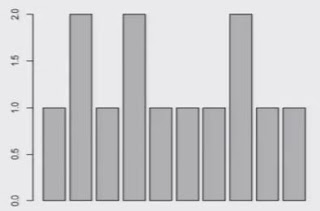# Statistical Functions - Graphics and Plots in R Language

Graphics tools :

Graphics tools - various type of plots
• 2D & 3D plots,
• scatter diagram
• Pie diagram
• Histogram
• Bar plot
• Stem and leaf plot
• Box plot ....
Appropriate number and choice of plots in analysis provides better inferences.

In R, such graphics can be easily created and saved in various formats.
• Bar plot
• Pie chart
• Box plot
• Grouped box plot
• Scatter plot
• Coplots
• Histogram
• Normal QQ plot ...

Bar plots :-

→ Visualize the relative or absolute frequencies of observed values of a variable.
→ It consists of one bar for each category.
→ The height of each bar is determined by either the absolute frequency or the relative frequency of the respective category and is shown on the y-axis.

barplot (x, width = 1, space = NULL ,...)
> barplot (table (x) )
> barplot (table (x) / length (x) )

Example :-
Code the 10 persons by using, say 1 for male (M) and 2 for female (F).
M, F, M, F, M, M, M, F, M, M
1,  2, 1,  2,  1,  1,   1,  2,  1,  1

> gender <-  c(1, 2, 1, 2, 1, 1, 1, 2, 1, 1)
> gender
  1  2  1  2  1  1  1  2  1  1Example :-
> barplot (gender)
Do you want this ?
2 categories
M = 7
F  = 3

Pie diagram :-

Pie charts visualize the absolute and relative frequencies.

A pie chart is a circle partitioned into segments where each of the segments represents a category.

The size of each segment depends upon the relative frequency and is determined by the angle (frequency x 360 degree).

pie (x,  labels  = names (x),  ...)

Example :-

> pie (gender)

Histogram :-

Histogram is based on the idea to categorize the data into different groups and plot the bars for each category with height.

The area of the bar (= height x width ) is proportional to the relative frequency.

So the width of the bars need not necessarily to be the same

hist (x)  # show absolute frequencies
hist (x, freq=F)   # show relative frequencies

see help ("hist") for more details